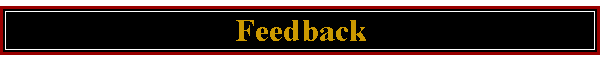#### <!-- msfphover = (((navigator.appname == "netscape") && (parseint(navigator.appversion) >= 3 )) || ((navigator.appname == "microsoft internet explorer") && (parseint(navigator.appversion) >= 4 ))); function msfppreload(img) { var a=new image(); a.src=img; return a; } // --><!-- if(msfphover) { msfpnav1n=msfppreload("_derived/ext1141_cmp_zero110_hbtn.gif"); msfpnav1h=msfppreload("_derived/ext1141_cmp_zero110_hbtn_a.gif"); } // -->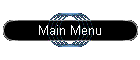<!-- if(msfphover) { msfpnav2n=msfppreload("_derived/up_cmp_zero110_hbtn.gif"); msfpnav2h=msfppreload("_derived/up_cmp_zero110_hbtn_a.gif"); } // -->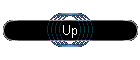<!-- if(msfphover) { msfpnav3n=msfppreload("_derived/aboutme.htm_cmp_zero110_hbtn.gif"); msfpnav3h=msfppreload("_derived/aboutme.htm_cmp_zero110_hbtn_a.gif"); } // -->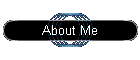<!-- if(msfphover) { msfpnav4n=msfppreload("_derived/table_of_contents.htm_cmp_zero110_hbtn.gif"); msfpnav4h=msfppreload("_derived/table_of_contents.htm_cmp_zero110_hbtn_a.gif"); } // -->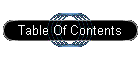<!-- if(msfphover) { msfpnav5n=msfppreload("_derived/site_map.htm_cmp_zero110_hbtn.gif"); msfpnav5h=msfppreload("_derived/site_map.htm_cmp_zero110_hbtn_a.gif"); } // -->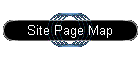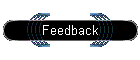<!-- if(msfphover) { msfpnav6n=msfppreload("_derived/search_1.htm_cmp_zero110_hbtn.gif"); msfpnav6h=msfppreload("_derived/search_1.htm_cmp_zero110_hbtn_a.gif"); } // -->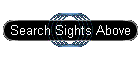<!-- if(msfphover) { msfpnav7n=msfppreload("_derived/copyright-privacy.htm_cmp_zero110_hbtn.gif"); msfpnav7h=msfppreload("_derived/copyright-privacy.htm_cmp_zero110_hbtn_a.gif"); } // -->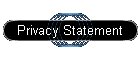tell me what you think about my web site.